Next: The Maple worksheet Up: Preliminaries about Maple Previous: Starting a Maple Session

Subsections

# Basic Maple

You can use Maple for numerical calculations, for symbolic manipulations, and for graphing purposes. All Maple commands must be ended with a semicolon ; and the corresponding stroke of the return key. To familiarize yourself with it, try to run the following examples and analyze the results. The commands that you type in and the Maple results are shown here in a way that is similar to what you see on the screen.

## Maple for numeric calculations

Most of the time you will be using Maple as a calculator. You can also write programs in Maple, but usually you will be using the tool expecting an immediate response. You type in your statement at the Maple prompt > and Maple responds with an answer:
>
1/2+3;The symbols for the basic operations are +, -, * and /. Exponentiation is denoted by ^:

>
77*(3^5+5^2)/(99-47);In the first example, the inverse of 2 is added to 3. In the second, we input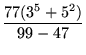, and Maple computes its value before printing the output.

You must always use the asterisk * for multiplication. Forgetting it in expressions like sin(2*x) or 4*x is easy, and may result in a syntax error message. Even if you don't get a syntax error, Maple will not treat the expression as you expect.

Maple works primarily with whole numbers or fractions. However, you can force it to write the decimal expansion of any number with evalf:

>
evalf(100*Pi);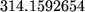>
b:=sqrt(2);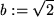>
evalf(b);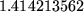>
evalf(b,47);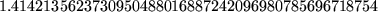Be careful with this example. The assignment of the variable b to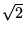is done with := rather than with =. This will be the case of any other assignment operation you do in Maple, as we discuss below. The equals sign = is used to signify that two things are equal, not to set one to the other.

Notice the difference in the following two examples:

>
sum(1/(2*i),i=1..10);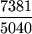>
sum(1.0/(2*i),i=1..10);In both cases, we compute(2i)-1. However, in the second case, the presence of 1.0 indicates to Maple the terms in this computation are approximate, and gives you a decimal expansion of the result.

## Maple for symbolic manipulations

We can ask Maple to give us a formula for the sum of the cube of the first n integers:
>
sum(i^3,i=1..n);We can ask to factor the resulting polynomial in n:

>
factor(In this example, Maple's ditto operator'' % is used1.3 to refer to the result previously obtained. Thus, in effect, the example above is equivalent to

>
factor(1/4*(n + 1)^4 - 1/2*(n + 1)^3 + 1/4*(n + 1)^2);One can expand or factor conveniently:

>
p:=(x+1)^4*(x-6)^3;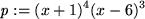>
expand(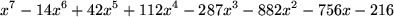>
factor(We can use Maple to solve equations (if b still has the valuefrom the previous section, first unassign it by executing the command b:='b'):

>>Notice that solve provides both roots of the quadratic polynomial.

Maple also divides polynomials. Here the command you execute has a syntax which is a little bit more complicated. Consider the following example:1.4

>
p:=x^10-1;
q:=x-1;
divide(p,q,b);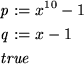We assign the polynomials x10 - 1 and x - 1 to the variables p and q, respectively, and then ask Maple to divide p by q.1.5Maple replies with the statement true to indicate that the polynomial q = x - 1 divides the polynomial p = x10 - 1 exactly, and places the quotient in the third argument of the divide command. Thus, if you want to know the value of the quotient, you must see what the variable b stands for now:

>
b;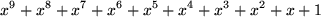Of course, the answer produced by divide will be false if the polynomials do not divide each other exactly. Then one can use the commands quo and rem to find the quotient and remainder of the division:

>
r:=x^2+2*x+3;>
divide(p,r,c);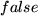>
quo(p,r,x);>
rem(p,r,x);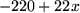We would have obtained an error if we would have tried divide(p,r,b), trying to place the quotient of the division in the variable b: it is no longer a variable since it has a value from the previous example:

>
divide(p,r,b);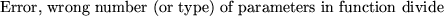The above command is equivalent to divide(p,r,x^9+x^8+x^7+x^6+x^5+x^4+x^3+x^2+x+1), which makes no sense at all. If we insist on calling the result of the division b, it would have been necessary to unassign the value of b first:

>
b:='b';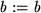We could also have done this within the command itself, using divide(p,r,'b').1.6Further discussion on this is given in section 4.2.

We can use solve for systems of equations. For example:

>
solve(1*x+(1/2)*y+(1/6)*z =1,
(1/2)*x+(1/6)*y+(1/24)*z =0,
(1/6)*x+(1/24)*y+(1/120)*z =1,  x,y,z );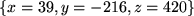Maple also solves inequalities:

>
solve(x^2-4*x-7>0,x);>
solve(abs(1-x^2)<1/2,x);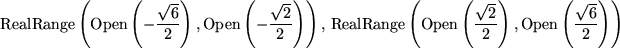## Graphing in Maple

Let us now use the graphics facilities of Maple. It is quite straightforward to draw the graph of a simple expression. For example, to graph the function sin(x2) over the range -3x3, we do the following.

>
plot(sin(x^2), x=-3..3);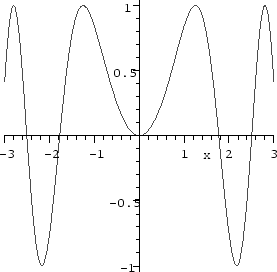Notice that within the worksheet interface, clicking the right mouse button on the displayed graph causes a menu to pop up that allows you to change some of its properties. Clicking the left mouse button causes a box to appear around the graph and the buttons on the toolbar to change. You can use the box to resize the graph, and the buttons to change attributes such as the style of the axes and the aspect ration.

In section 9 we shall discuss in further detail the graphing features of Maple.

#### Footnotes

... used1.3
In Maple V release 4 and earlier, Maple used the double-quote " to refer to the result of the previous command instead of %. If you encounter an older Maple program, you might have to change this, among some other things.
... example:1.4
In the worksheet interface, we can insert linebreaks by holding the shift key and the return key simultaneously. Maple does not execute what we type until we hit the return key unshifted.
... q.1.5
Of course, we could have done all of this in a single Maple command divide(x^10-1, x-1, b); if we had no intention of using p or q again.
... divide(p,r,'b').1.6
In both cases, it is very important to use the proper quotation marks; using " or  means a very different thing, and would not work. The quotation mark used here is the single quote' or `right quote', and on most keyboards is on the same key as the double quoute, near the enter key.Next: The Maple worksheet Up: Preliminaries about Maple Previous: Starting a Maple Session

Translated from LaTeX by Scott Sutherland
2002-08-29